Geometry 8-3 Trigonometry
starstarstarstarstarstarstarstarstarstar
3 (1 rating)
by Matthew Richardson
| 26 Questions
Note from the author:
A complete formative lesson with embedded slideshow, mini lecture screencasts, checks for understanding, practice items, mixed review, and reflection. I create these assignments to supplement each lesson of Pearson's Common Core Edition Algebra 1, Algebra 2, and Geometry courses. See also mathquest.net and twitter.com/mathquestEDU.
The outlined content above was added from outside of Formative.
The outlined content above was added from outside of Formative.1
1
10 pts
Solve It! What is the ratio of the length of the shorter leg to the length of the hypotenuse for △ADF, AEG, and △ABC? Not all ratios will be used.
• 5/6
• (√5)/6
• (√5)/5
• △AEG
• △ABC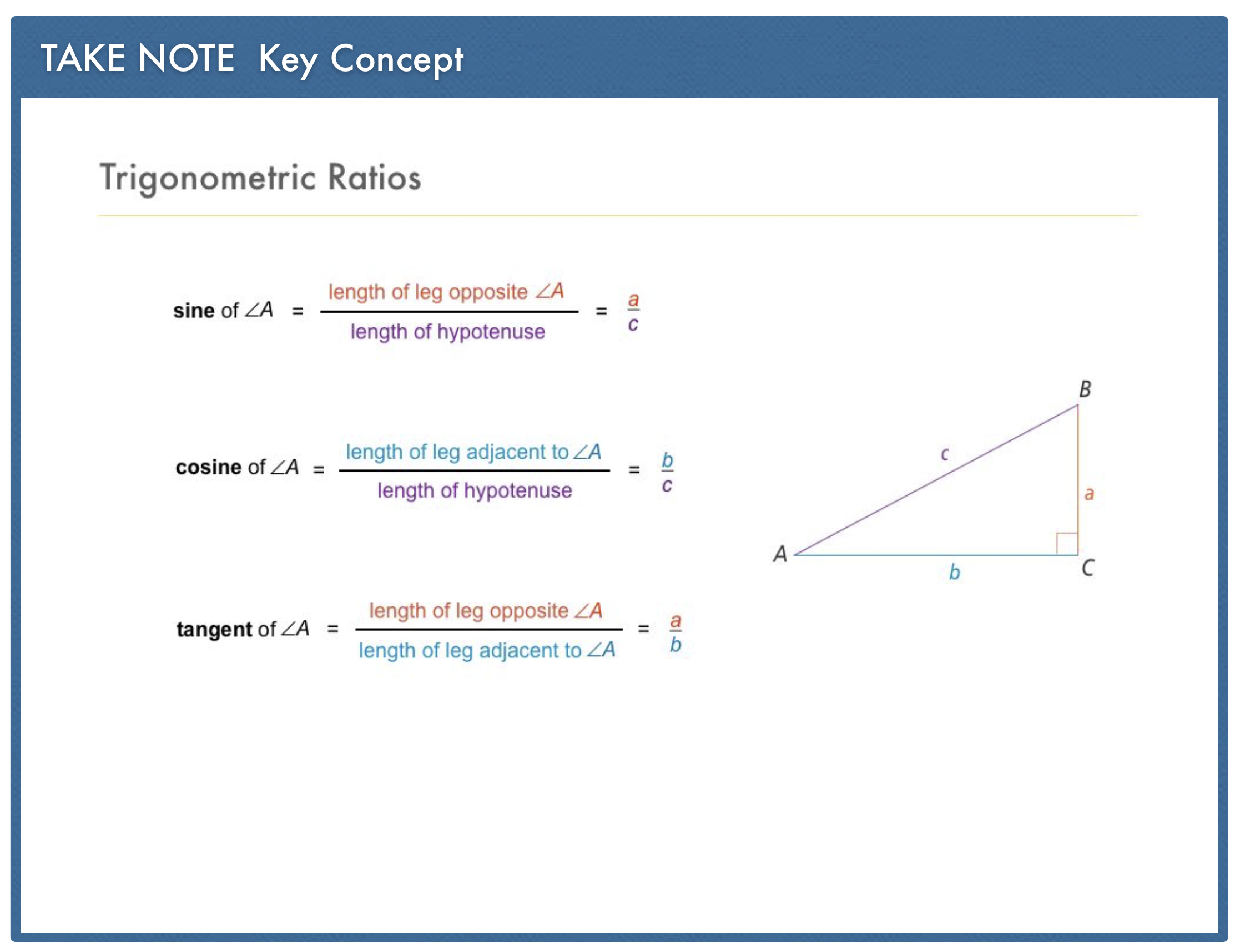The outlined content above was added from outside of Formative.2
2
10 pts
Problem 1 Got It?
A
B
C
D
The outlined content above was added from outside of Formative.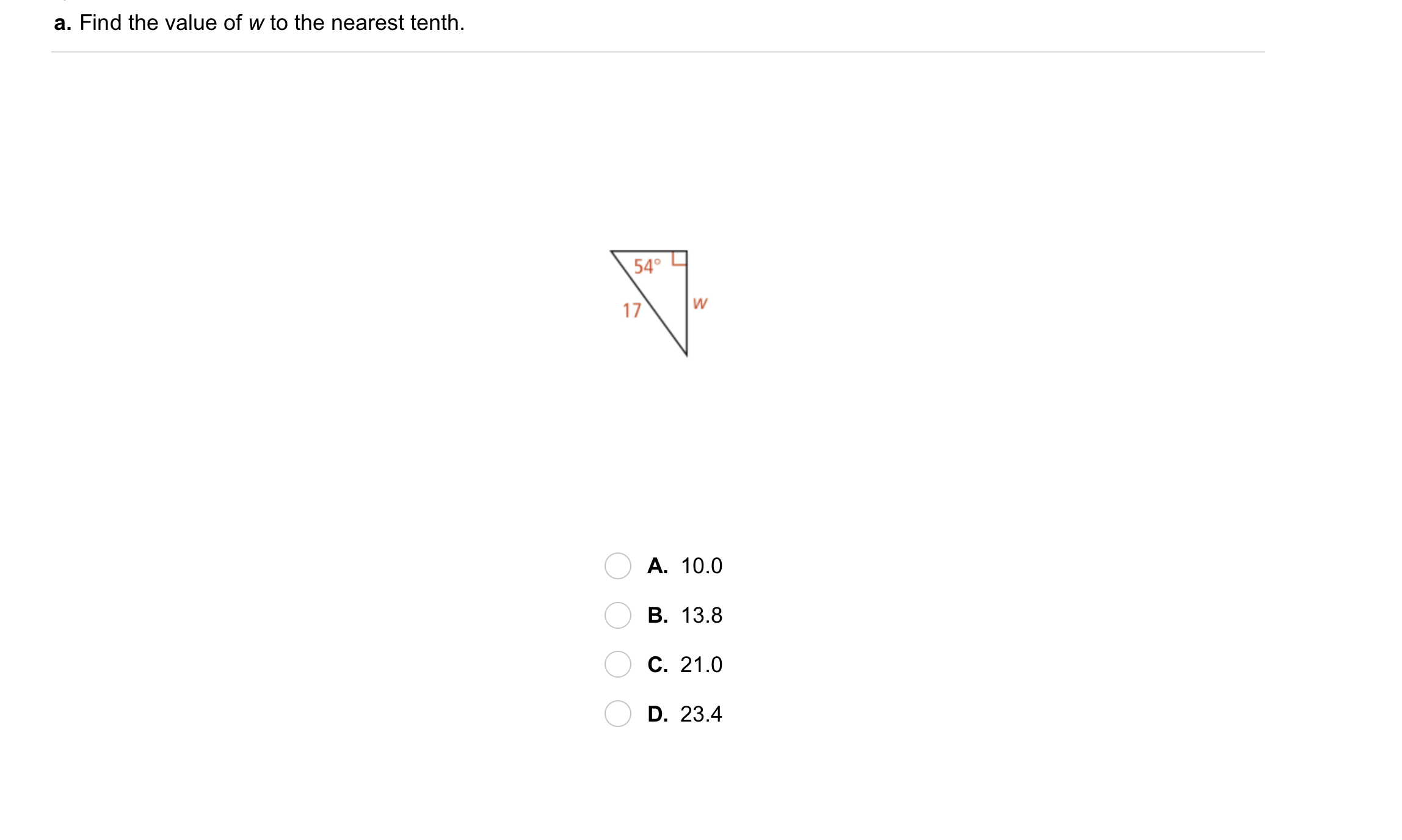3
3
10 pts
Problem 2 Got It?
A
B
C
D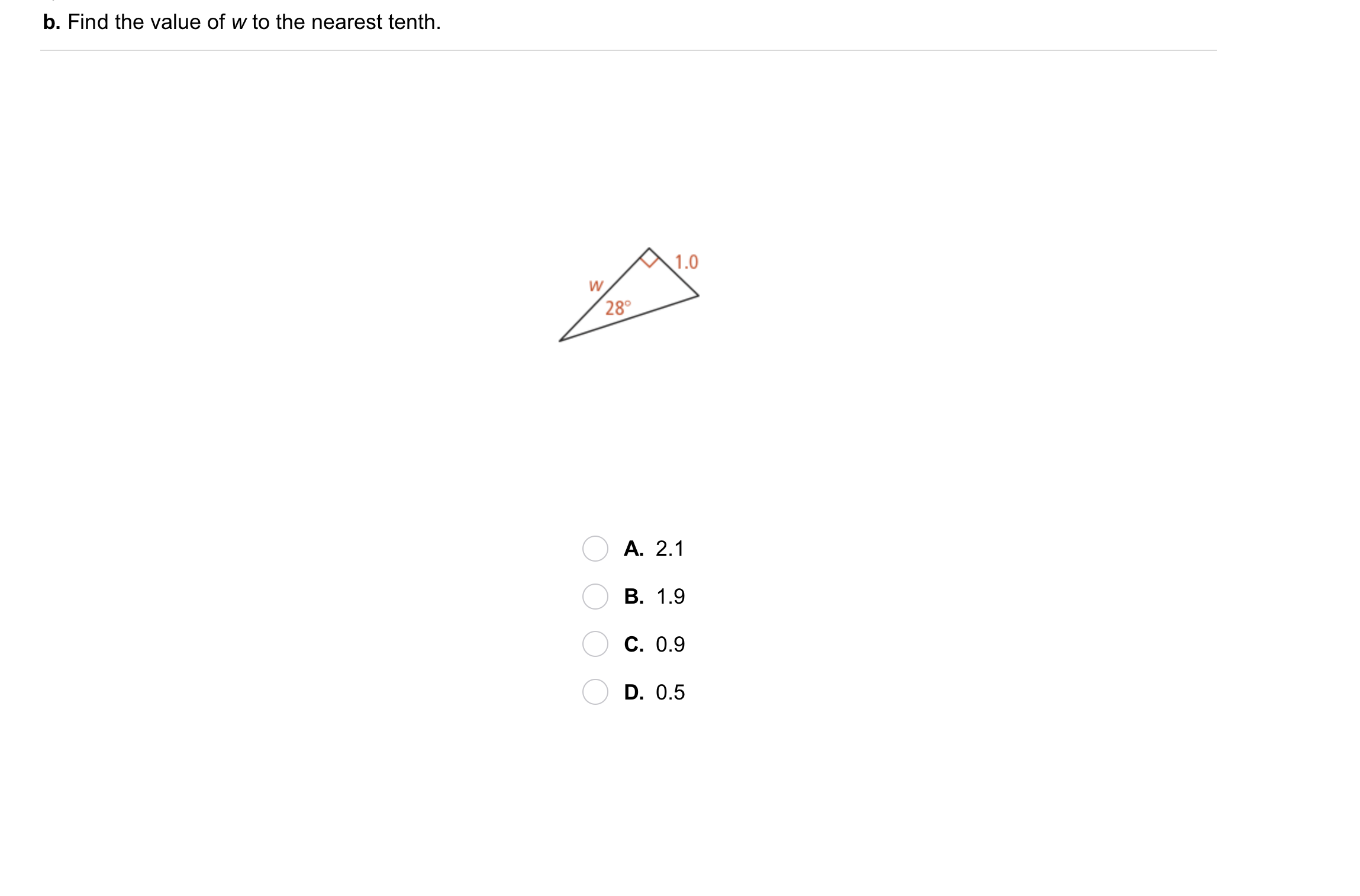4
4
10 pts
Problem 2 Got It?
A
B
C
D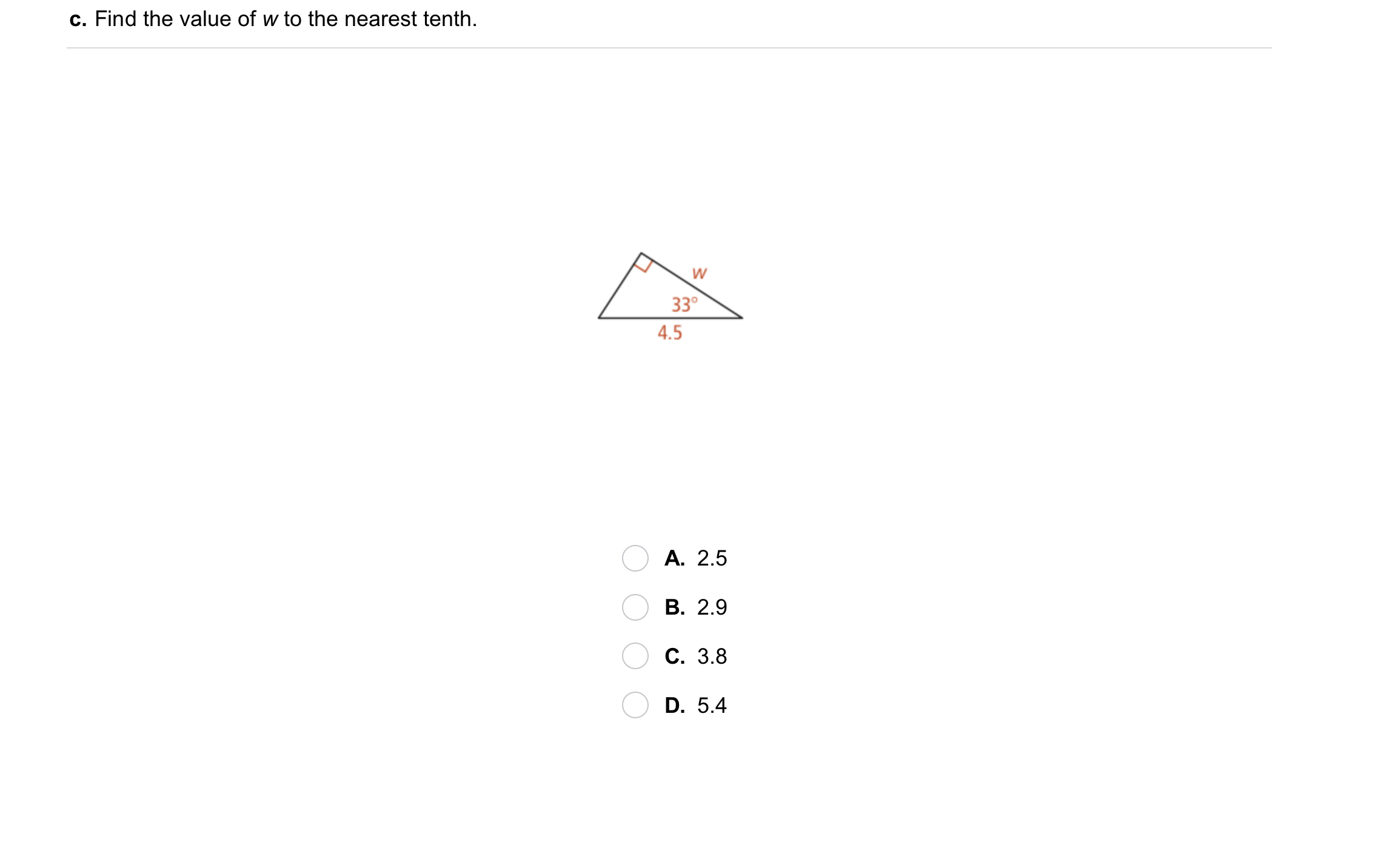5
5
10 pts
Problem 2 Got It?
A
B
C
D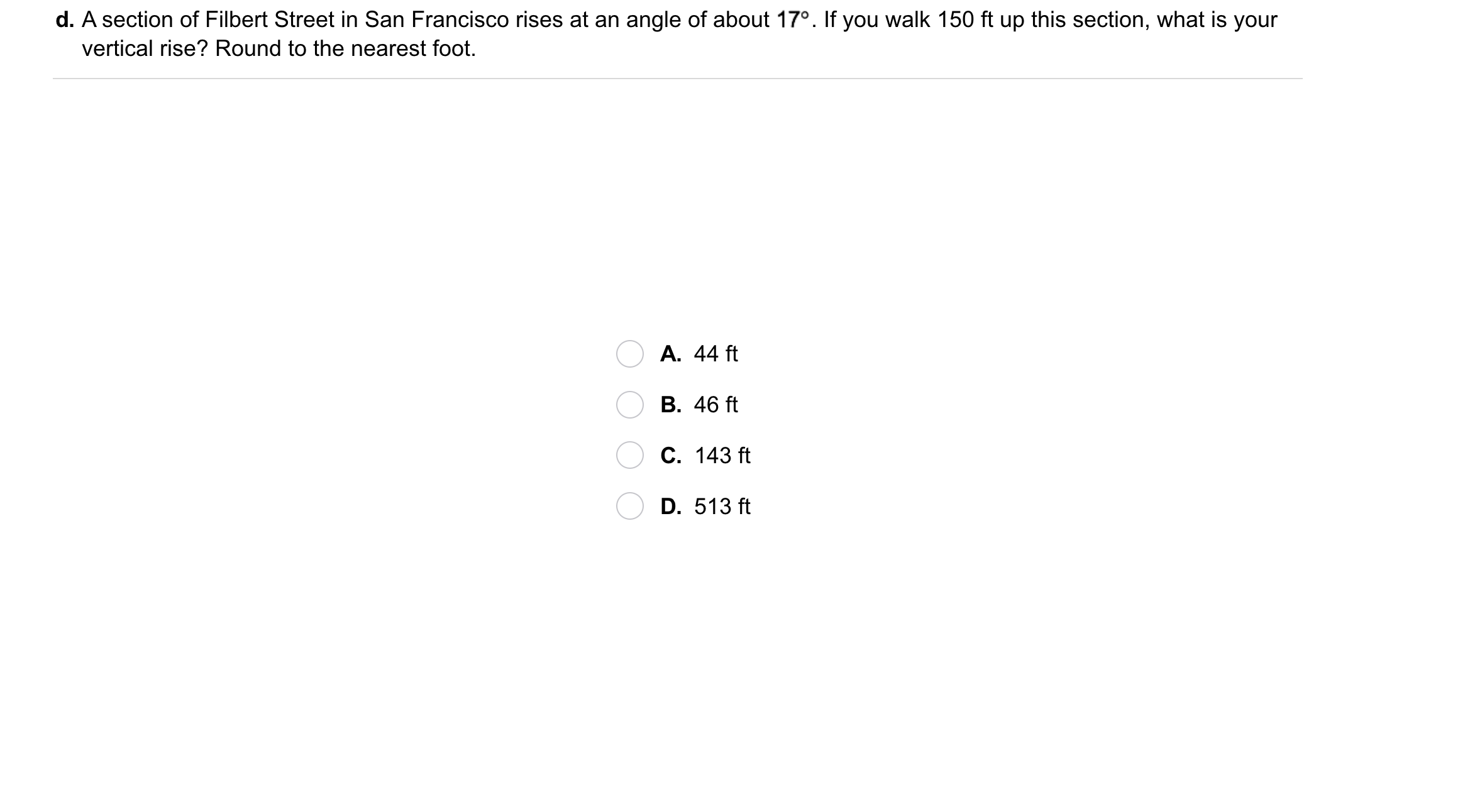6
6
10 pts
Problem 2 Got It?
A
B
C
DThe outlined content above was added from outside of Formative.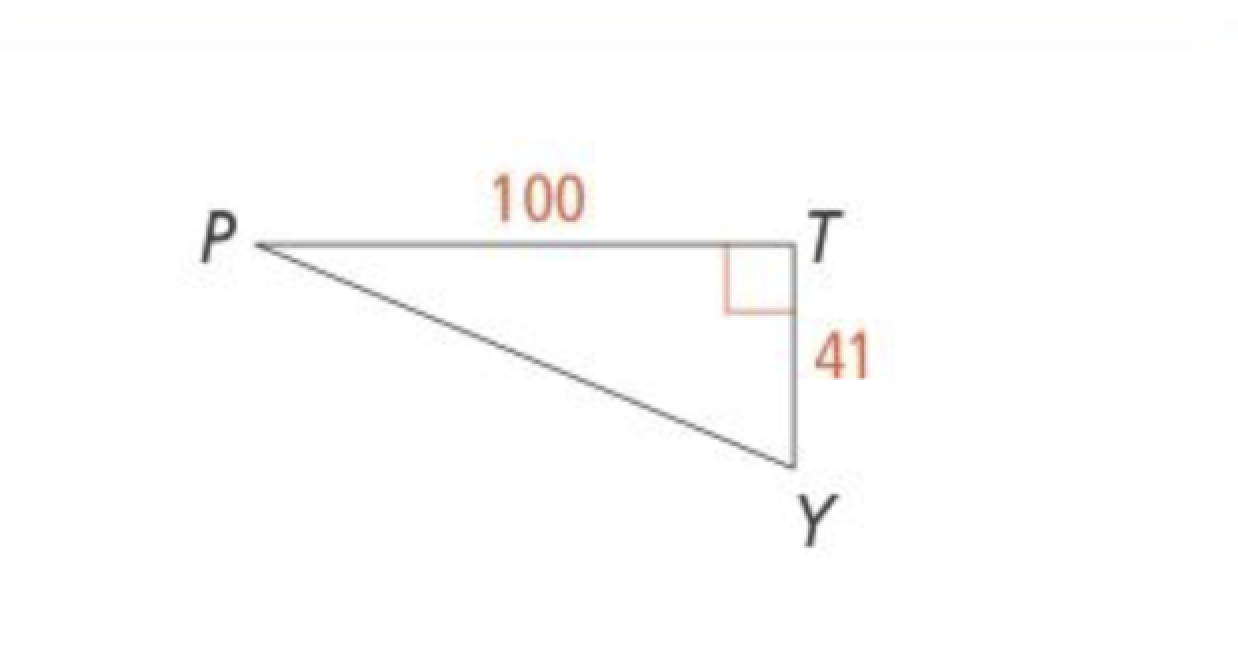7
7
10 pts
Problem 3 Got It? Use the figure in the diagram. What is m∠Y to the nearest degree? Enter only a number.
8
10 pts
Problem 3 Got It? Reasoning: Suppose you know the lengths of all three sides of a right triangle. Does it matter which trigonometric ratio you use to find the measures of any of the three angles? Explain.9
9
10 pts
A
B
C
D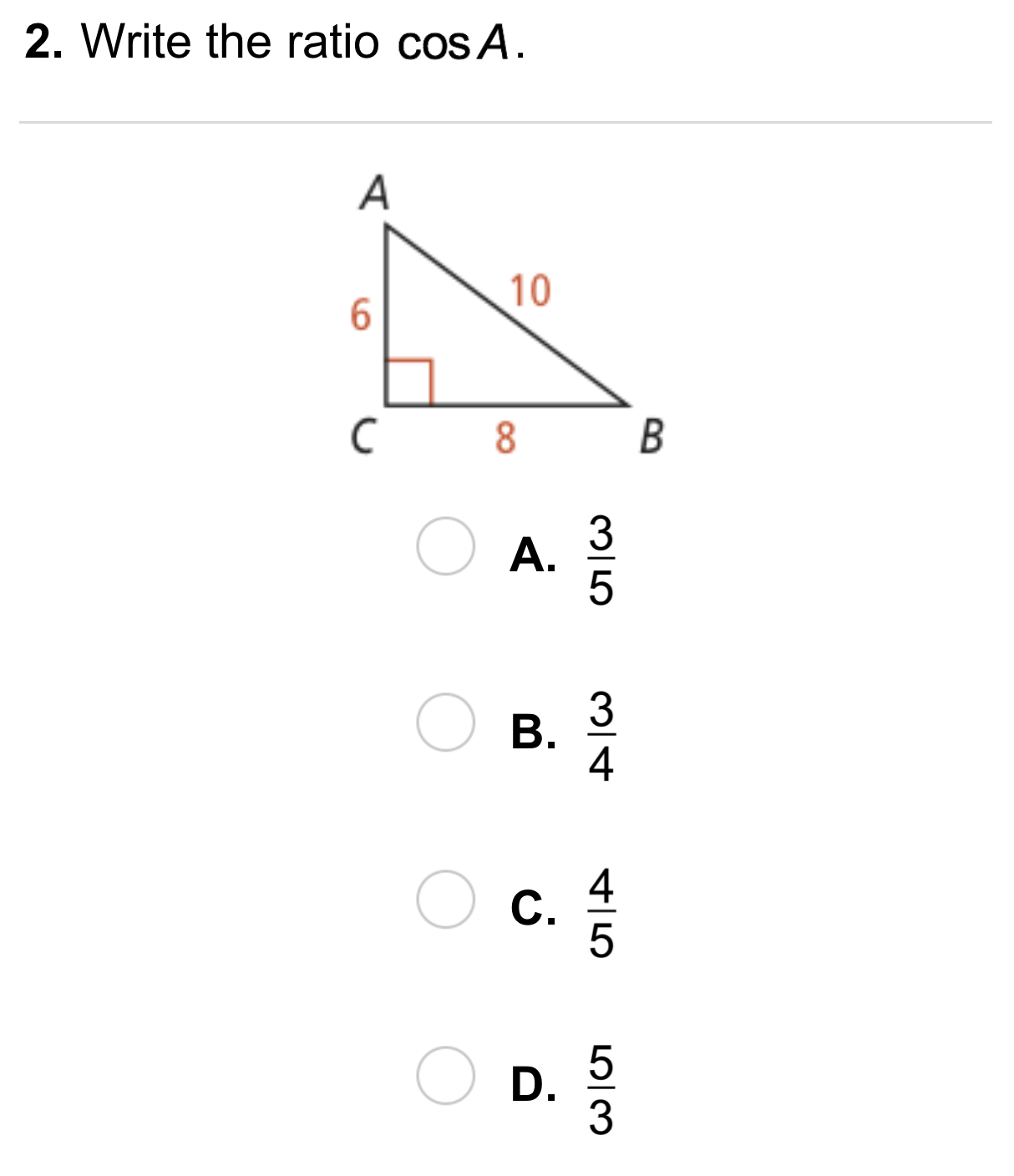10
10
10 pts
A
B
C
D11
11
10 pts
A
B
C
D12
12
10 pts
A
B
C
D13
13
10 pts
A
B
C
D14
14
10 pts
A
B
C
D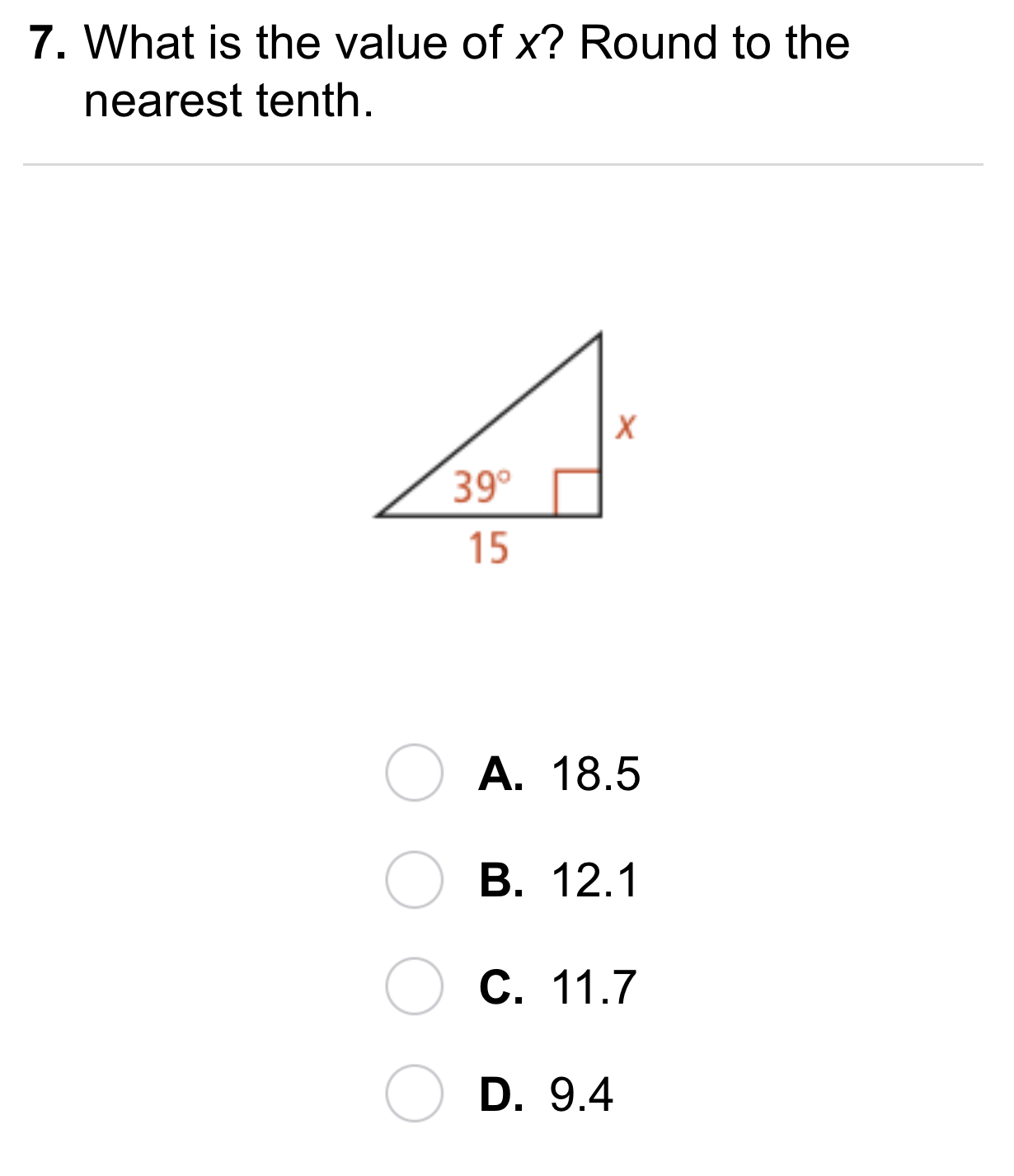15
15
10 pts
A
B
C
D16
16
10 pts
A
B
C
D18
18
10 pts
Error Analysis: A student states that sin A > sin X because the lengths of the sides of △ABC are greater than the lengths of △XYZ. What is the student's error? Explain.19
10 pts
Review Lesson 8-2: The length of the hypotenuse of a 30-60-90 triangle is 8. What are the lengths of the legs? Select all that apply.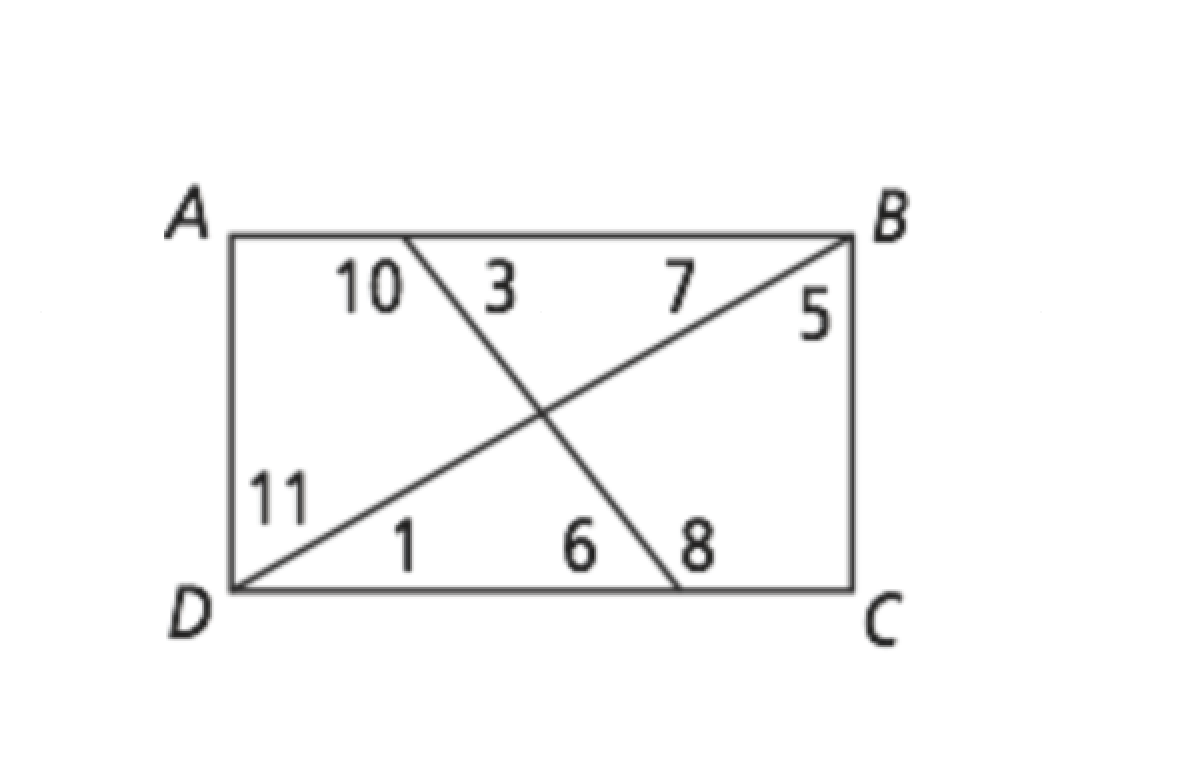20
20
10 pts
Review Lessons 3-2 & 6-4: Use rectangle ABCD to match congruent angles.
• ∠1
• ∠5
• ∠3
• ∠6
• ∠7
• ∠11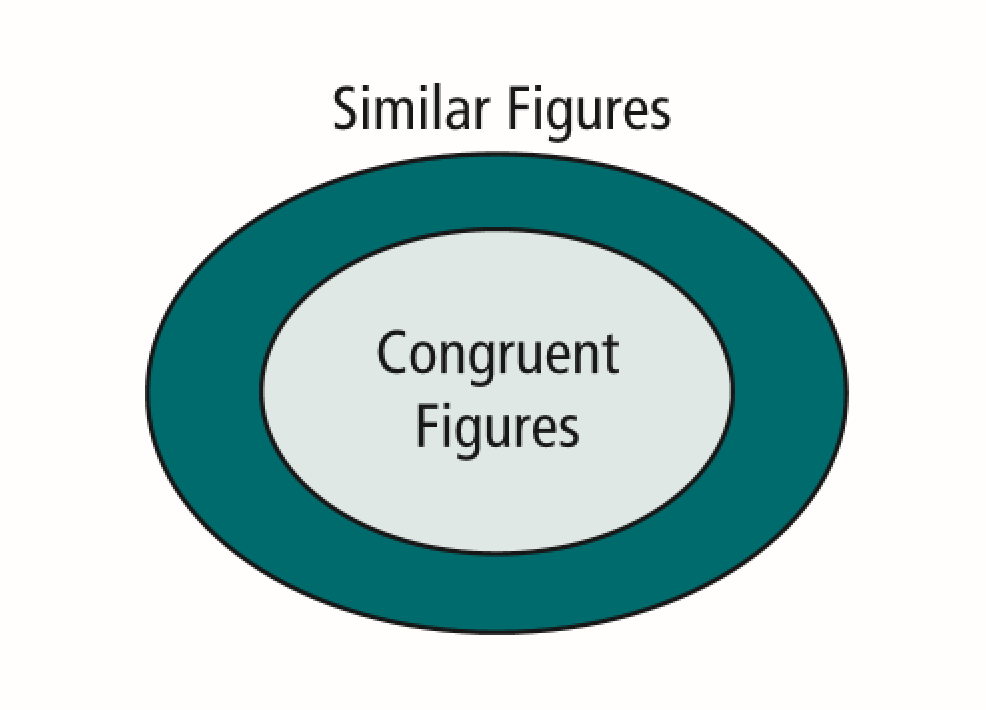21
21
10 pts
Vocabulary Review: The Venn diagram shows the relationship between similar and congruent figures. Label each statement as True or False.
• True
• False
• All similar figures are congruent figures.
• All congruent figures are similar figures.
• Some similar figures are congruent figures.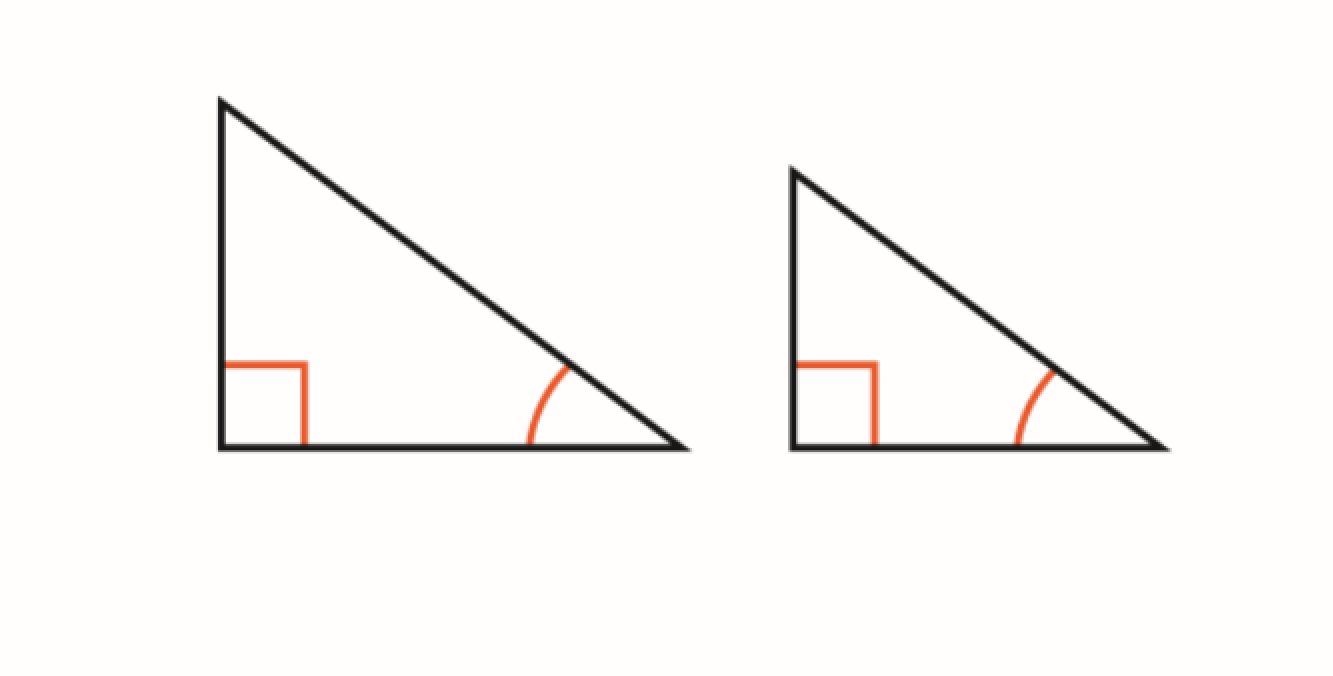22
22
10 pts
Vocabulary Review: Which postulate or theorem can you use to verify that the triangles in the diagram are similar.
AA ~ Postulate
SAS ~ Theorem
SSS ~ Theorem23
23
10 pts
Use Your Vocabulary: Identify the ratio of the length of the longer leg to the length of the shorter leg.
5/13
5/12
12/13
13/12
12/5
13/5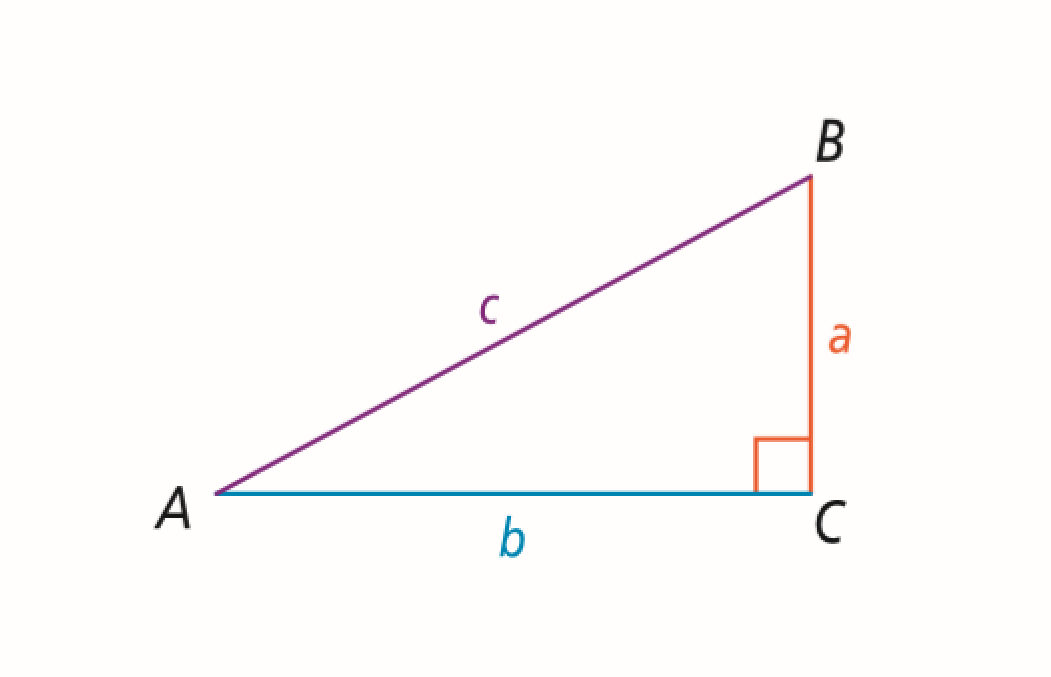24
24
10 pts
Use Your Vocabulary: Match each trigonometric ratio with its corresponding side length ratio.
• sin B
• cos B
• tan B
• a/c
• b/a
• b/c
25
10 pts
Reasoning: Suppose △ABC is a right isosceles triangle. What would the tangent of ∠B equal? Enter only a number.
26
10 pts
Reflection: Math Success
Add to my formatives list

Formative uses cookies to allow us to better understand how the site is used. By continuing to use this site, you consent to the Terms of Service and Privacy Policy.